Anúncio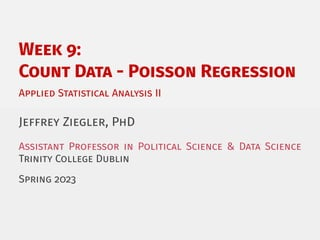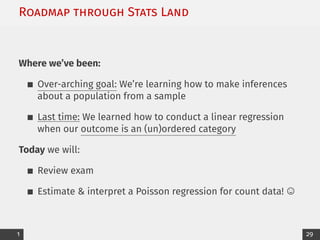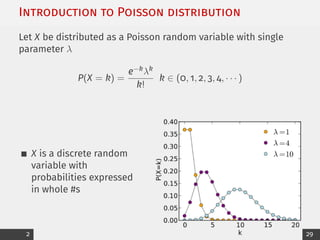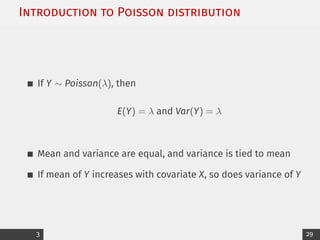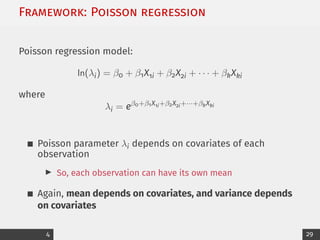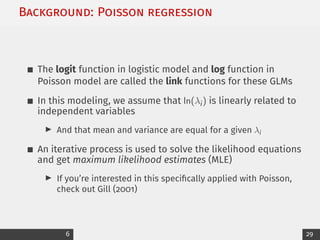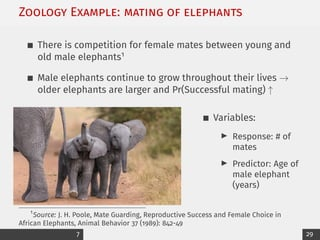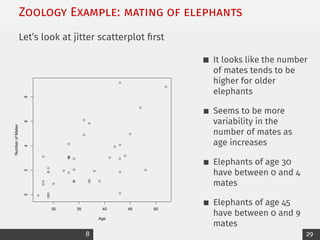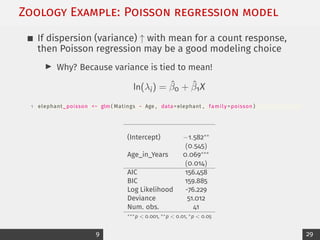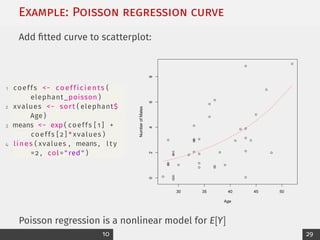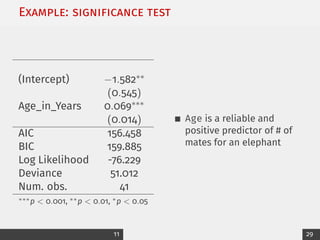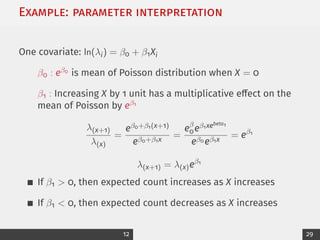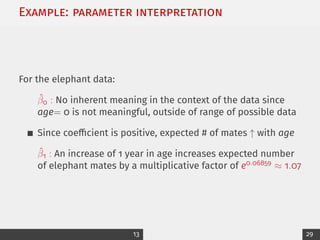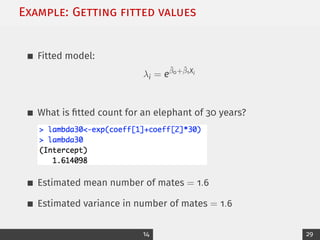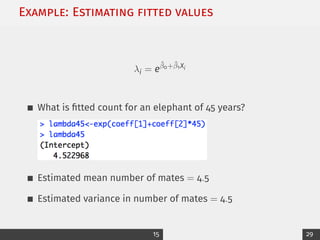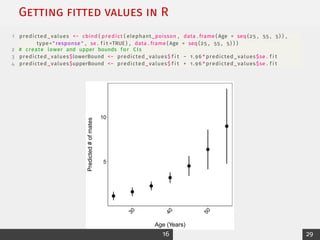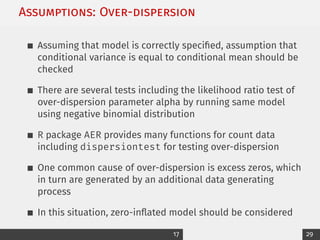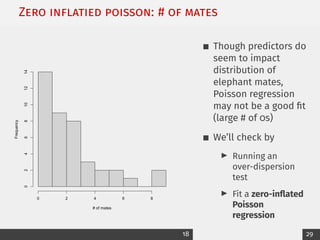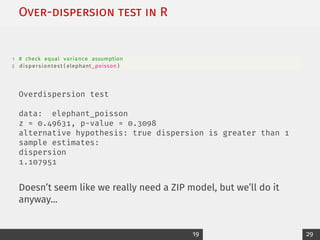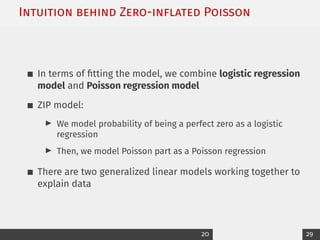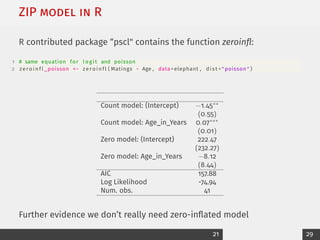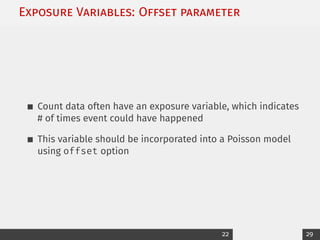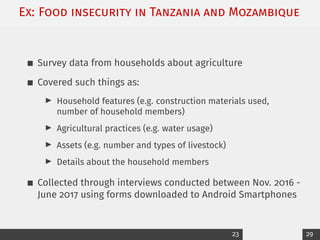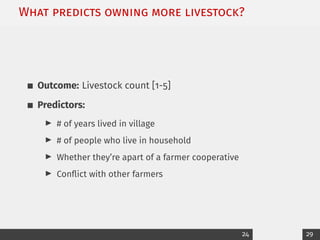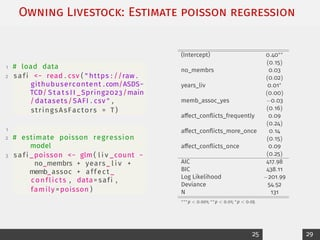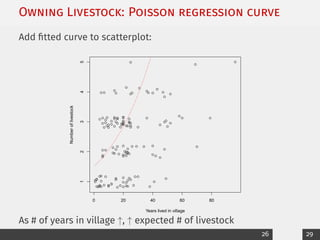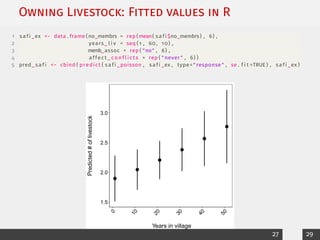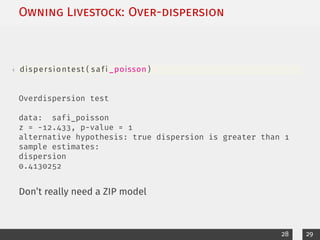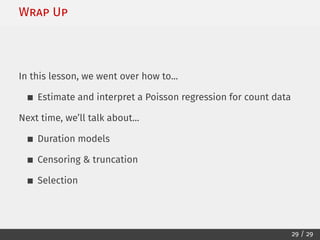1 de 30
Anúncio

### 9_Poisson_printable.pdf

1. Week 9: Count Data - Poisson Regression Applied Statistical Analysis II Jeffrey Ziegler, PhD Assistant Professor in Political Science & Data Science Trinity College Dublin Spring 2023
2. Roadmap through Stats Land Where we’ve been: Over-arching goal: We’re learning how to make inferences about a population from a sample Last time: We learned how to conduct a linear regression when our outcome is an (un)ordered category Today we will: Review exam Estimate & interpret a Poisson regression for count data! © 1 29
3. Introduction to Poisson distribution Let X be distributed as a Poisson random variable with single parameter λ P(X = k) = e−kλk k! k ∈ (0, 1, 2, 3, 4, · · · ) X is a discrete random variable with probabilities expressed in whole #s 2 29
4. Introduction to Poisson distribution If Y ∼ Poisson(λ), then E(Y) = λ and Var(Y) = λ Mean and variance are equal, and variance is tied to mean If mean of Y increases with covariate X, so does variance of Y 3 29
5. Framework: Poisson regression Poisson regression model: ln(λi) = β0 + β1X1i + β2X2i + · · · + βkXki where λi = eβ0+β1X1i+β2X2i+···+βkXki Poisson parameter λi depends on covariates of each observation I So, each observation can have its own mean Again, mean depends on covariates, and variance depends on covariates 4 29
6. Background: Poisson regression Poisson regression is another generalized linear model Instead of a log function of Bernoulli parameter πi (logistic regression), we use a log function of Poisson parameter λi λi > 0 → −∞ < ln(λi) < ∞ 5 29
7. Background: Poisson regression The logit function in logistic model and log function in Poisson model are called the link functions for these GLMs In this modeling, we assume that ln(λi) is linearly related to independent variables I And that mean and variance are equal for a given λi An iterative process is used to solve the likelihood equations and get maximum likelihood estimates (MLE) I If you’re interested in this specifically applied with Poisson, check out Gill (2001) 6 29
8. Zoology Example: mating of elephants There is competition for female mates between young and old male elephants1 Male elephants continue to grow throughout their lives → older elephants are larger and Pr(Successful mating) ↑ Variables: I Response: # of mates I Predictor: Age of male elephant (years) 1 Source: J. H. Poole, Mate Guarding, Reproductive Success and Female Choice in African Elephants, Animal Behavior 37 (1989): 842-49 7 29
9. Zoology Example: mating of elephants Let’s look at jitter scatterplot first 30 35 40 45 50 0 2 4 6 8 Age Number of Mates It looks like the number of mates tends to be higher for older elephants Seems to be more variability in the number of mates as age increases Elephants of age 30 have between 0 and 4 mates Elephants of age 45 have between 0 and 9 mates 8 29
10. Zoology Example: Poisson regression model If dispersion (variance) ↑ with mean for a count response, then Poisson regression may be a good modeling choice I Why? Because variance is tied to mean! ln(λi) = β̂0 + β̂1X 1 elephant_poisson <− glm ( Matings ~ Age , data=elephant , family =poisson ) (Intercept) −1.582∗∗ (0.545) Age_in_Years 0.069∗∗∗ (0.014) AIC 156.458 BIC 159.885 Log Likelihood -76.229 Deviance 51.012 Num. obs. 41 ∗∗∗p < 0.001, ∗∗p < 0.01, ∗p < 0.05 9 29
11. Example: Poisson regression curve Add fitted curve to scatterplot: 1 coeffs <− coefficients ( elephant_poisson ) 2 xvalues <− sort ( elephant\$ Age ) 3 means <− exp ( coeffs [ 1 ] + coeffs [ 2 ] * xvalues ) 4 lines ( xvalues , means , l t y =2 , col = " red " ) 30 35 40 45 50 0 2 4 6 8 Age Number of Mates Poisson regression is a nonlinear model for E[Y] 10 29
12. Example: signiﬁcance test (Intercept) −1.582∗∗ (0.545) Age_in_Years 0.069∗∗∗ (0.014) AIC 156.458 BIC 159.885 Log Likelihood -76.229 Deviance 51.012 Num. obs. 41 ∗∗∗p < 0.001, ∗∗p < 0.01, ∗p < 0.05 Age is a reliable and positive predictor of # of mates for an elephant 11 29
13. Example: parameter interpretation One covariate: ln(λi) = β0 + β1Xi β0 : eβ0 is mean of Poisson distribution when X = 0 β1 : Increasing X by 1 unit has a multiplicative effect on the mean of Poisson by eβ1 λ(x+1) λ(x) = eβ0+β1(x+1) eβ0+β1x = eβ 0eβ1xebeta1 eβ0 eβ1x = eβ1 λ(x+1) = λ(x)eβ1 If β1 > 0, then expected count increases as X increases If β1 < 0, then expected count decreases as X increases 12 29
14. Example: parameter interpretation For the elephant data: β̂0 : No inherent meaning in the context of the data since age= 0 is not meaningful, outside of range of possible data Since coefficient is positive, expected # of mates ↑ with age β̂1 : An increase of 1 year in age increases expected number of elephant mates by a multiplicative factor of e0.06859 ≈ 1.07 13 29
15. Example: Getting ﬁtted values Fitted model: λi = eβ̂0+β̂1Xi What is fitted count for an elephant of 30 years? Estimated mean number of mates = 1.6 Estimated variance in number of mates = 1.6 14 29
16. Example: Estimating ﬁtted values λi = eβ̂0+β̂1Xi What is fitted count for an elephant of 45 years? Estimated mean number of mates = 4.5 Estimated variance in number of mates = 4.5 15 29
17. Getting ﬁtted values in R 1 predicted_values <− cbind ( predict ( elephant_poisson , data . frame ( Age = seq (25 , 55 , 5) ) , type=" response " , se . f i t =TRUE ) , data . frame ( Age = seq (25 , 55 , 5) ) ) 2 # create lower and upper bounds for CIs 3 predicted_values\$lowerBound <− predicted_values\$ f i t − 1.96 * predicted_values\$se . f i t 4 predicted_values\$upperBound <− predicted_values\$ f i t + 1.96 * predicted_values\$se . f i t 5 10 3 0 4 0 5 0 Age (Years) Predicted # of mates 16 29
18. Assumptions: Over-dispersion Assuming that model is correctly specified, assumption that conditional variance is equal to conditional mean should be checked There are several tests including the likelihood ratio test of over-dispersion parameter alpha by running same model using negative binomial distribution R package AER provides many functions for count data including dispersiontest for testing over-dispersion One common cause of over-dispersion is excess zeros, which in turn are generated by an additional data generating process In this situation, zero-inflated model should be considered 17 29
19. Zero inﬂatied poisson: # of mates # of mates Frequency 0 2 4 6 8 0 2 4 6 8 10 12 14 Though predictors do seem to impact distribution of elephant mates, Poisson regression may not be a good fit (large # of 0s) We’ll check by I Running an over-dispersion test I Fit a zero-inflated Poisson regression 18 29
20. Over-dispersion test in R 1 # check equal variance assumption 2 dispersiontest ( elephant_poisson ) Overdispersion test data: elephant_poisson z = 0.49631, p-value = 0.3098 alternative hypothesis: true dispersion is greater than 1 sample estimates: dispersion 1.107951 Doesn’t seem like we really need a ZIP model, but we’ll do it anyway... 19 29
21. Intuition behind Zero-inﬂated Poisson In terms of fitting the model, we combine logistic regression model and Poisson regression model ZIP model: I We model probability of being a perfect zero as a logistic regression I Then, we model Poisson part as a Poisson regression There are two generalized linear models working together to explain data 20 29
22. ZIP model in R R contributed package “pscl" contains the function zeroinfl: 1 # same equation for l o g i t and poisson 2 z e r o i n f l _poisson <− z e r o i n f l ( Matings ~ Age , data=elephant , dist =" poisson " ) Count model: (Intercept) −1.45∗∗ (0.55) Count model: Age_in_Years 0.07∗∗∗ (0.01) Zero model: (Intercept) 222.47 (232.27) Zero model: Age_in_Years −8.12 (8.44) AIC 157.88 Log Likelihood -74.94 Num. obs. 41 Further evidence we don’t really need zero-inflated model 21 29
23. Exposure Variables: Offset parameter Count data often have an exposure variable, which indicates # of times event could have happened This variable should be incorporated into a Poisson model using offset option 22 29
24. Ex: Food insecurity in Tanzania and Mozambique Survey data from households about agriculture Covered such things as: I Household features (e.g. construction materials used, number of household members) I Agricultural practices (e.g. water usage) I Assets (e.g. number and types of livestock) I Details about the household members Collected through interviews conducted between Nov. 2016 - June 2017 using forms downloaded to Android Smartphones 23 29
25. What predicts owning more livestock? Outcome: Livestock count [1-5] Predictors: I # of years lived in village I # of people who live in household I Whether they’re apart of a farmer cooperative I Conflict with other farmers 24 29
26. Owning Livestock: Estimate poisson regression 1 # load data 2 s a f i <− read . csv ( " https : //raw . githubusercontent . com/ASDS− TCD/ S t a t s I I _Spring2023/main /datasets/SAFI . csv " , stringsAsFactors = T ) 1 2 # estimate poisson regression model 3 s a f i _poisson <− glm ( l i v _count ~ no_membrs + years_ l i v + memb_assoc + affect _ conflicts , data= safi , family =poisson ) (Intercept) 0.40∗∗ (0.15) no_membrs 0.03 (0.02) years_liv 0.01∗ (0.00) memb_assoc_yes −0.03 (0.16) affect_conflicts_frequently 0.09 (0.24) affect_conflicts_more_once 0.14 (0.15) affect_conflicts_once 0.09 (0.25) AIC 417.98 BIC 438.11 Log Likelihood −201.99 Deviance 54.52 N 131 ∗∗∗p < 0.001; ∗∗p < 0.01; ∗p < 0.05 25 29
27. Owning Livestock: Poisson regression curve Add fitted curve to scatterplot: 0 20 40 60 80 1 2 3 4 5 Years lived in village Number of livestock As # of years in village ↑, ↑ expected # of livestock 26 29
28. Owning Livestock: Fitted values in R 1 s a f i _ex <− data . frame (no_membrs = rep (mean( s a f i \$no_membrs) , 6) , 2 years_ l i v = seq ( 1 , 60 , 10) , 3 memb_assoc = rep ( "no" , 6) , 4 affect _ c o n f l i c t s = rep ( " never " , 6) ) 5 pred_ s a f i <− cbind ( predict ( s a f i _poisson , s a f i _ex , type= " response " , se . f i t =TRUE ) , s a f i _ex ) 1.5 2.0 2.5 3.0 0 1 0 2 0 3 0 4 0 5 0 Years in village Predicted # of livestock 27 29
29. Owning Livestock: Over-dispersion 1 dispersiontest ( s a f i _poisson ) Overdispersion test data: safi_poisson z = -12.433, p-value = 1 alternative hypothesis: true dispersion is greater than 1 sample estimates: dispersion 0.4130252 Don’t really need a ZIP model 28 29
30. Wrap Up In this lesson, we went over how to... Estimate and interpret a Poisson regression for count data Next time, we’ll talk about... Duration models Censoring & truncation Selection 29 / 29
Anúncio